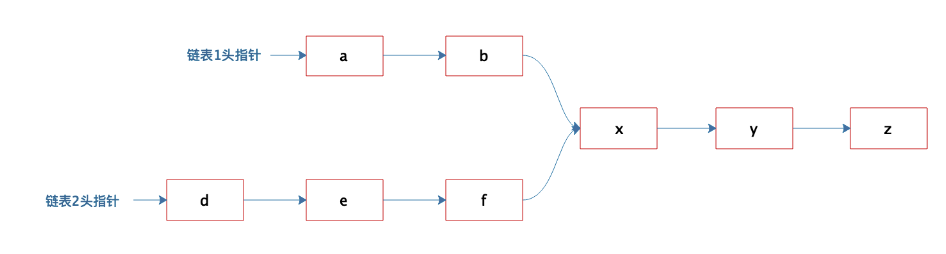# 算法题：链表的第一个合并节点﻿

1. 得到两个链表的长度

2. 长的链表头指针移动一个长度差。接着，两个链表的指针同时向下移动，如果指向同一个节点，则该节点为合并的节点。

class Node(object):    """链表中的节点"""    def __init__(self, content, next_node):        self.content = content        self.next = next_node        def __str__(self):        if self.next:            return "%s->%s" % (self.content, self.next)        else:            return "%s->%s" % (self.content, None)def create_link(list_1, list_2, merge_list):    """创建链表    list1 = list_1 + merge_list    list2 = list_2 + merge_list    """    merge_node = add_nodes(merge_list, None)    print "merge_node: ", merge_node    list_1_first = add_nodes(list_1, merge_node)    print "list_1_first", list_1_first    list_2_first = add_nodes(list_2, merge_node)    print "list_2_first", list_2_first    return list_1_first, list_2_first            def add_nodes(list_num, last_node):    """给定链表头，在链表头之前，加入新的元素    list_num: 需要插入的元素    last_node: 插入的链表的头    """    now_next = last_node    if list_num:        for i in xrange(len(list_num), 0, -1):            temp_node = Node(list_num[i-1], now_next)            now_next = temp_node        return temp_node      else:        return now_next

def find_merge_node(start_node_x, start_node_y):    """    给定链表头，发现两个链表是否合并？不合并返回None，合并返回合并的第一个元素。    """    len_x = get_node_len(start_node_x)    len_y = get_node_len(start_node_y)    if len_x > len_y:        long_node = start_node_x        short_node = start_node_y    else:        long_node = start_node_y        short_node = start_node_x        for _ in xrange(0, abs(len_x - len_y)):        long_node = long_node.next        while long_node and short_node:        if long_node == short_node:            return long_node        long_node = long_node.next        short_node = short_node.next    return Nonedef get_node_len(start_node):    """获得链表长度"""    link_len = 0    temp_node = start_node    while temp_node:        link_len += 1        temp_node = temp_node.next        return link_len

link_1, link_2 = create_link(list_1=['a', 'b'], list_2=['d','e', 'f'], merge_list=['x', 'y', 'z'])merge_node = find_merge_node(link_1, link_2)print "result:", merge_node.content

result: x

﻿## 评论